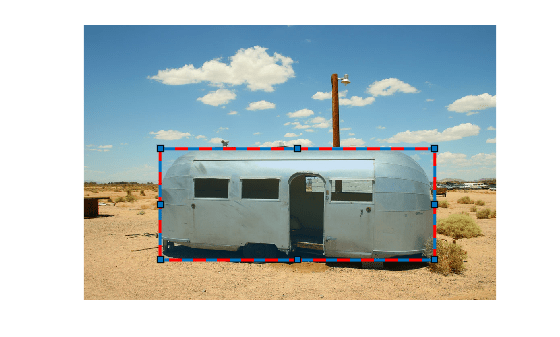# inROI

Query if points are located in ROI

## Syntax

``tf = inROI(ROI,x,y)``
``tf = inROI(ROI,x,y,z)``

## Description

example

``` `tf = inROI(ROI,x,y)` returns a logical array, `tf`, that indicates whether points with coordinates (`x`,`y`) are inside or outside the ROI.```
``` `tf = inROI(ROI,x,y,z)` returns a logical array, `tf`, that indicates whether points with coordinates (`x`,`y`,`z`) are inside or outside the `Cuboid` ROI.```

## Examples

collapse all

Read an image into the workspace and display it.

```I = imread('trailer.jpg'); figure imshow(I)```

Draw a rectangular ROI on the image, using the `Position` argument to specify the position of the rectangle as `[xmin,ymin,width,height]`.

`h = drawrectangle('Position',[190 308 682 276],'StripeColor','r');`Specify the x- and y-coordinates of three points. The last point is the upper left corner of the rectangular ROI.

```xcoords = [335 335 190]; ycoords = [200 400 308];```

Query if the three points are inside the ROI.

`tf = inROI(h,xcoords,ycoords)`
```tf = 3x1 logical array 0 1 1 ```

## Input Arguments

collapse all

Region of interest, specified as an ROI object of one of the following types:

X-coordinates of the query points, specified as a numeric scalar or vector.

Y-coordinates of the query points, specified as a numeric scalar or vector.

Y-coordinates of the query points, specified as a numeric scalar or vector.

## Output Arguments

collapse all

Status of query points, returned as a logical array. The array is the same length as the input arrays `x`, `y`, and `z`. Elements of the logical array set to `true` indicate that the corresponding query point is inside the ROI. Elements that are `false` indicate the point is not inside the ROI.